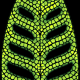Script Drawing :

• DrawLine – Draws a line on a texture – optional stroke
• Paint – Paints with custom colour at a position on a texture
• PaintLine – Same as Paint but draws along a line
• DrawBezier – Draws a bezier curve on a texture – This function is very slow and takes at least 1 second do perform
• Sample – Returns an anti-aliasing pattern from a specified position

Script Mathfx:

• Rotate Vector – Rotates a Vector2 a number of degrees
• Normalize – Normalizes a Vector2
• IntersectPoint – Calculates the point two lines will intersect
• ThreePointCircle – Calculates the centre point of a circle passing through three points.
• NearestPointOnCircle – Calculates the nearest point on a circle
• CubicBezier – Calculates a point on a bezier curve from T (0-1)
• NearestPointOnBezier – Calculates the nearest point on a bezier curve
• NearestPointOnBezier – Same as above but takes four points instead of a BezierCurve class – Slower
• IsNearBezierTest – Is a point in a certain distance from the bezier curve (true/false)
• IsNearBezier – Same as above but takes two BezierPoints instead of a BezierCurve, the above is recommended
• IsNearBeziers – Loops through a number of BezierPoints and calculates if a point is near any of them (assumes all points is connected with BezierPoints)

Script GUIControls:

• RGBSlider – Makes an auto-layout RGB slider
• RGBCircle – Creates an RGB colour circle similar to Apples built-in – Needs a background image coming with the project

1.Nitin Dhami says:

2.3.